The UCM Procedure

IRREGULAR Statement

Subsections:

• IRREGULAR <options>;

The IRREGULAR statement includes an irregular component in the model. There can be at most one IRREGULAR statement in the model specification. The irregular component corresponds to the overall random error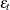in the model. By default the irregular component is modeled as white noise—that is, as a sequence of independent, identically distributed, zero-mean, Gaussian random variables. However, you can also model it as an autoregressive moving average (ARMA) process. The options for specifying an ARMA model for the irregular component are given in a separate subsection: ARMA Specification.

The options in this statement enable you to specify the model for the irregular component and to output its estimates. Two examples of the IRREGULAR statement are given next. In the first example the statement is in its simplest form, resulting in the inclusion of an irregular component that is white noise with unknown variance:

   irregular;


The following statement provides a starting value for the white noise variance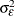to be used in the nonlinear parameter estimation process. It also requests the printing of smoothed estimates of. The smoothed irregulars are useful in model diagnostics.

   irregular variance=4 print=smooth;

NOEST

fixes the value ofto the value specified in the VARIANCE= option. Also see the NOEST= option in the subsection ARMA Specification.

PLOT=FILTER
PLOT=SMOOTH
PLOT=( <FILTER> <SMOOTH> )

requests plotting of the filtered or smoothed estimate of the irregular component.

PRINT=FILTER
PRINT=SMOOTH
PRINT=( <FILTER> <SMOOTH> )

requests printing of the filtered or smoothed estimate of the irregular component.

VARIANCE=value

specifies an initial value forduring the parameter estimation process. Any nonnegative value, including zero, is an acceptable starting value.

ARMA Specification

This section details the options for specifying an ARMA model for the irregular component. The specification of ARMA models requires some notation, which is explained first.

Let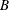denote the backshift operator—that is, for any sequence,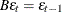. The higher powers ofrepresent larger shifts (for example,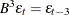). A random sequencefollows a zero-mean ARMA(p,q)(P,Q)model with nonseasonal autoregressive order p, seasonal autoregressive order P, nonseasonal moving average order q, and seasonal moving average order Q, if it satisfies the following difference equation specified in terms of the polynomials in the backshift operator where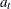is a white noise sequence and s is the season length: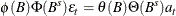The polynomials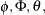and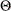are of orders p, P, q, and Q, respectively, which can be any nonnegative integers. The season length s must be a positive integer. For example,satisfies an ARMA(1,1) model (that is,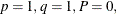and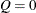) if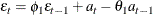for some coefficients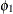and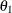and a white noise sequence. Similarlysatisfies an ARMA(1,1)(1,1)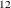model if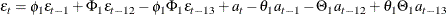for some coefficients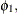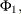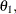and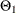and a white noise sequence. The ARMA process is stationary and invertible if the defining polynomialsandhave all their roots outside the unit circle—that is, their absolute values are strictly larger than 1.0. It is assumed that the ARMA model specified for the irregular component is stationary and invertible—that is, the coefficients of the polynomialsandare constrained so that the stationarity and invertibility conditions are satisfied. The unknown coefficients of these polynomials become part of the model parameter vector that is estimated using the data.

The notation for a closely related class of models, autoregressive integrated moving average (ARIMA) models, is also given here. A random sequence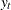is said to follow an ARIMA(p,d,q)(P,D,Q)model if, for some nonnegative integers d and D, the differenced series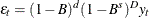follows an ARMA(p,q)(P,Q)model. The integers d and D are called nonseasonal and seasonal differencing orders, respectively. You can specify ARIMA models by using the DEPLAG statement for specifying the differencing orders and by using the IRREGULAR statement for the ARMA specification. See Example 34.8 for an example of ARIMA(0,1,1)(0,1,1)model specification. Brockwell and Davis (1991) can be consulted for additional information about ARIMA models.

You can use options of the IRREGULAR statement to specify the desired ARMA model and to request printed and graphical output. A few examples of the IRREGULAR statement are given next.

The following statement specifies an irregular component that is modeled as an ARMA(1,1) process. It also requests plotting its smoothed estimate.

   irregular p=1 q=1 plot=smooth;


The following statement specifies an ARMA(1,1)(1,1)model. It also fixes the coefficient of the first-order seasonal moving average polynomial to 0.1. The other coefficients and the white noise variance are estimated using the data.

   irregular p=1 sp=1 q=1 sq=1 s=12 sma=0.1 noest=(sma);


AR=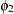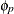lists the starting values of the coefficients of the nonseasonal autoregressive polynomial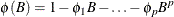where the order p is specified in the P= option. The coefficients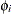must define a stationary autoregressive polynomial.

MA=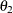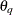lists the starting values of the coefficients of the nonseasonal moving average polynomial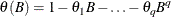where the order q is specified in the Q= option. The coefficients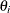must define an invertible moving average polynomial.

NOEST=(<VARIANCE> <AR> <SAR> <MA> <SMA>)

fixes the values of the ARMA parameters and the value of the white noise variance to those specified in the AR= , SAR= , MA= , SMA= , or VARIANCE= options.

P=integer

specifies the order of the nonseasonal autoregressive polynomial. The order can be any nonnegative integer; the default value is 0. In practice the order is a small integer such as 1, 2, or 3.

Q=integer

specifies the order of the nonseasonal moving average polynomial. The order can be any nonnegative integer; the default value is 0. In practice the order is a small integer such as 1, 2, or 3.

S=integer

specifies the season length used during the specification of the seasonal autoregressive or seasonal moving average polynomial. The season length can be any positive integer; for example, S=4 might be an appropriate value for a quarterly series. The default value is S=1.

SAR=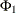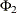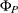lists the starting values of the coefficients of the seasonal autoregressive polynomial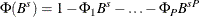where the order P is specified in the SP= option and the season length s is specified in the S= option. The coefficients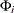must define a stationary autoregressive polynomial.

SMA=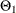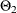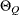lists the starting values of the coefficients of the seasonal moving average polynomial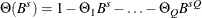where the order Q is specified in the SQ= option and the season length s is specified in the S= option. The coefficients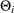must define an invertible moving average polynomial.

SP=integer

specifies the order of the seasonal autoregressive polynomial. The order can be any nonnegative integer; the default value is 0. In practice the order is a small integer such as 1 or 2.

SQ=integer

specifies the order of the seasonal moving average polynomial. The order can be any nonnegative integer; the default value is 0. In practice the order is a small integer such as 1 or 2.